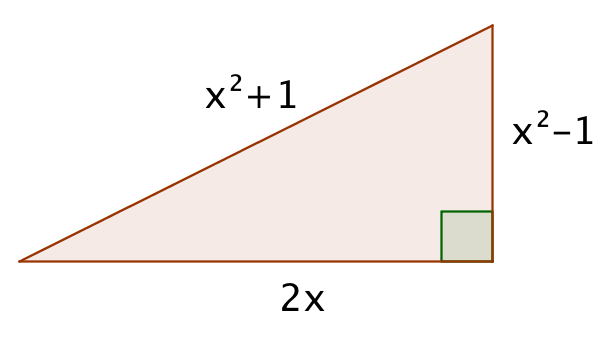# Polynomials

## Objective

Use polynomial identities to determine Pythagorean triples.

## Common Core Standards

### Core Standards

?

• A.APR.C.4 — Prove polynomial identities and use them to describe numerical relationships. For example, the polynomial identity (x² + y²)2 = (x² — y²)² + (2xy)² can be used to generate Pythagorean triples.

?

• 8.G.B.7

## Criteria for Success

?

1. Describe the general rule for identifying Pythagorean triples, and show examples that it works.
2. Describe that by repeatedly identifying the same numerical relationship, you can develop an identity for a known pattern.
3. Deconstruct a set of Pythagorean triples into the polynomial identity.
4. Generate Pythagorean triples using the identity.

## Tips for Teachers

?

Ensure that students memorize the following sets of Pythagorean triples: 3-4-5, 5-12-13, and 7-24-25.

## Anchor Problems

?

### Problem 1

Prove that if ${ x>1}$, then a triangle with side lengths ${x^2-1}$, ${2x}$, and ${x^2+1}$  is a right triangle.#### Guiding Questions

Create a free account or sign in to access the Guiding Questions for this Anchor Problem.

#### References

EngageNY Mathematics Algebra II > Module 1 > Topic A > Lesson 10Example 1

Algebra II > Module 1 > Topic A > Lesson 10 of the New York State Common Core Mathematics Curriculum from EngageNY and Great Minds. © 2015 Great Minds. Licensed by EngageNY of the New York State Education Department under the CC BY-NC-SA 3.0 US license. Accessed Dec. 2, 2016, 5:15 p.m..

### Problem 2

A triangle has side lengths of ${(x^2-y^2 )}$, ${(2xy)}$, and ${(x^2+y^2 )}$. Choose values for $x$ and $y$. Will the resultant three sides be a right triangle? How do you know?

#### Guiding Questions

Create a free account or sign in to access the Guiding Questions for this Anchor Problem.

## Problem Set

?

The following resources include problems and activities aligned to the objective of the lesson that can be used to create your own problem set.

• Include problems where students are asked to identify the value of $x$ and $y$ within a Pythagorean triples pattern.

## Target Task

?

### Problem 1

Generate three Pythagorean triples

### Problem 2

Describe, algebraically, how you know that${(x^2-y^2)}$, ${(2xy)}$, and ${(x^2+y^2)}$ will always result in side lengths for a right triangle.